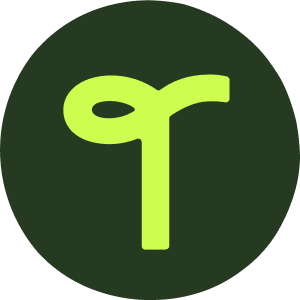teaching resource

Equal Groups - Hands-On Activity

A hands-on activity to practice making equal groups to represent multiplication.

Use this resource when teaching about equal groups when representing multiplication.

In this activity, students will model making equal groups using manipulatives, then record information regarding their model.

More comprehensive instructions on how to play are included in the resource.

Print the mat and cards on cardstock, and cut out the cards. Place the mat in a dry-erase sleeve so that students can use a dry-erase marker to wipe clean after each use.

This resource makes a great math center activity for students to play! It is also great to use with your guided math groups.

Use the drop-down menu to choose between the color or black and white version.

Looking for a set of paper manipulatives to use with this resource? Click on the link below for a set of colored bear counters.

Colored Bear Counters

A template including 70 colored bear counters.1 pageGrades: PK - 3

Curriculum

• CCSS.MATH.CONTENT.2.OA.C.4

Use addition to find the total number of objects arranged in rectangular arrays with up to 5 rows and up to 5 columns; write an equation to express the total as a sum of equal addends.

• CCSS.MATH.CONTENT.3.OA.B.5

Apply properties of operations as strategies to multiply and divide.2 Examples: If 6 × 4 = 24 is known, then 4 × 6 = 24 is also known. (Commutative property of multiplication.) 3 × 5 × 2 can be found by 3 × 5 = 15, then 15 × 2 = 30, or by 5 × ...